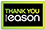Free Delivery on orders over €10

# Theory and Applications of Abstract Semilinear Cauchy Problems

Category: Mathematics
Several types of differential equations, such as functional differential equation, age-structured models, transport equations, reaction-diffusion equations, and partial differential equations with delay, can be formulated as abstract Cauchy problems with non-dense domain. This monograph provides a self-contained and comprehensive presentation of the fundamental theory of non-densely defined semilinear Cauchy problems and their applications. Starting from the classical Hille-Yosida theorem, semigroup method, and spectral theory, this monograph introduces the abstract Cauchy problems with non-dense domain, integrated semigroups, the existence of integrated solutions, positivity of solutions, Lipschitz perturbation, differentiability of solutions with respect to the state variable, and time differentiability of solutions. Combining the functional analysis method and bifurcation approach in dynamical systems, then the nonlinear dynamics such as the stability of equilibria, center manifold theory, Hopf bifurcation, and normal form theory are established for abstract Cauchy problems with non-dense domain. Finally applications to functional differential equations, age-structured models, and parabolic equations are presented. This monograph will be very valuable for graduate students and researchers in the fields of abstract Cauchy problems, infinite dimensional dynamical systems, and their applications in biological, chemical, medical, and physical problems.
€153.99461 Reward Points
In stock online
Delivery in 5 - 7 working days

Any purchases for more than €10 are eligible for free delivery anywhere in the UK or Ireland!

Several types of differential equations, such as functional differential equation, age-structured models, transport equations, reaction-diffusion equations, and partial differential equations with delay, can be formulated as abstract Cauchy problems with non-dense domain. This monograph provides a self-contained and comprehensive presentation of the fundamental theory of non-densely defined semilinear Cauchy problems and their applications. Starting from the classical Hille-Yosida theorem, semigroup method, and spectral theory, this monograph introduces the abstract Cauchy problems with non-dense domain, integrated semigroups, the existence of integrated solutions, positivity of solutions, Lipschitz perturbation, differentiability of solutions with respect to the state variable, and time differentiability of solutions. Combining the functional analysis method and bifurcation approach in dynamical systems, then the nonlinear dynamics such as the stability of equilibria, center manifold theory, Hopf bifurcation, and normal form theory are established for abstract Cauchy problems with non-dense domain. Finally applications to functional differential equations, age-structured models, and parabolic equations are presented. This monograph will be very valuable for graduate students and researchers in the fields of abstract Cauchy problems, infinite dimensional dynamical systems, and their applications in biological, chemical, medical, and physical problems.
Quantity:
In stock online
Delivery in 5 - 7 working days461 Reward Points

Any purchases for more than €10 are eligible for free delivery anywhere in the UK or Ireland!

€153.99
In stock online
Delivery in 5 - 7 working days
Quantity:461 Reward Points

Any purchases for more than €10 are eligible for free delivery anywhere in the UK or Ireland!

### Product Description

Several types of differential equations, such as functional differential equation, age-structured models, transport equations, reaction-diffusion equations, and partial differential equations with delay, can be formulated as abstract Cauchy problems with non-dense domain. This monograph provides a self-contained and comprehensive presentation of the fundamental theory of non-densely defined semilinear Cauchy problems and their applications. Starting from the classical Hille-Yosida theorem, semigroup method, and spectral theory, this monograph introduces the abstract Cauchy problems with non-dense domain, integrated semigroups, the existence of integrated solutions, positivity of solutions, Lipschitz perturbation, differentiability of solutions with respect to the state variable, and time differentiability of solutions. Combining the functional analysis method and bifurcation approach in dynamical systems, then the nonlinear dynamics such as the stability of equilibria, center manifold theory, Hopf bifurcation, and normal form theory are established for abstract Cauchy problems with non-dense domain. Finally applications to functional differential equations, age-structured models, and parabolic equations are presented. This monograph will be very valuable for graduate students and researchers in the fields of abstract Cauchy problems, infinite dimensional dynamical systems, and their applications in biological, chemical, medical, and physical problems.

### Product Details

#### Theory and Applications of Abstract Semilinear Cauchy Problems

ISBN9783030015053

Format

PublisherSPRINGER (03 December. 2018)

No. of Pages543

Weight1010

Language English (United States)

Dimensions 235 x 155 x 33